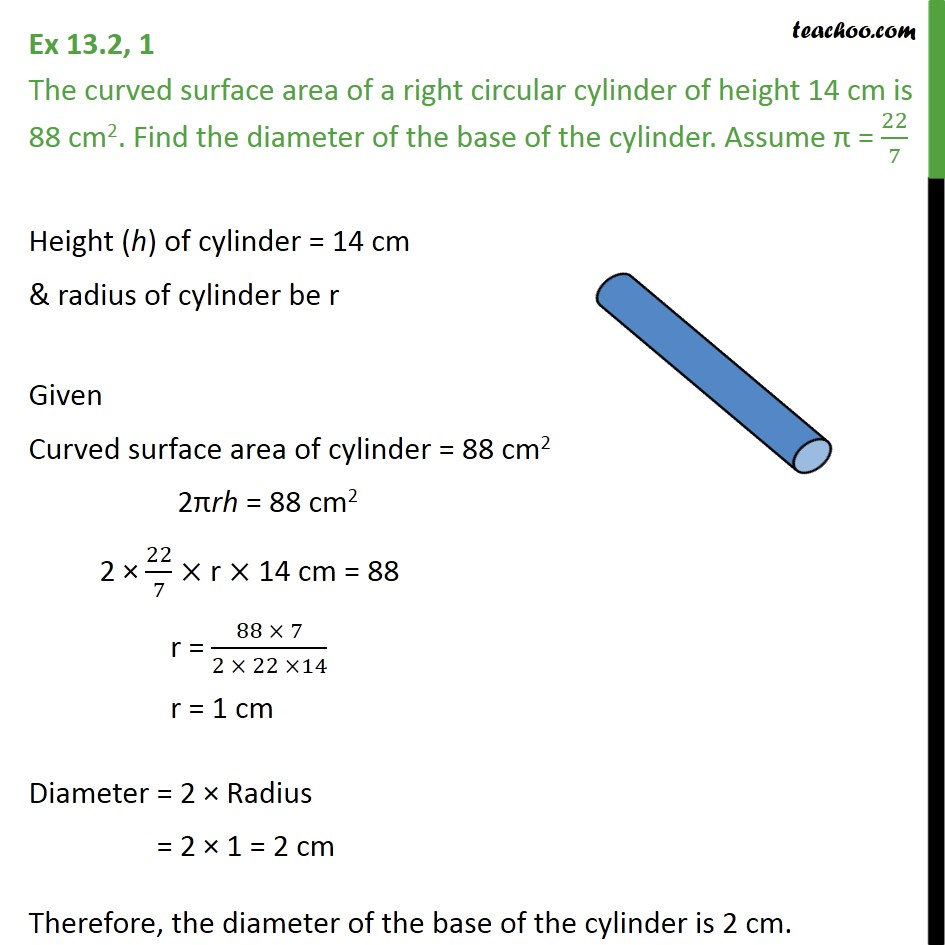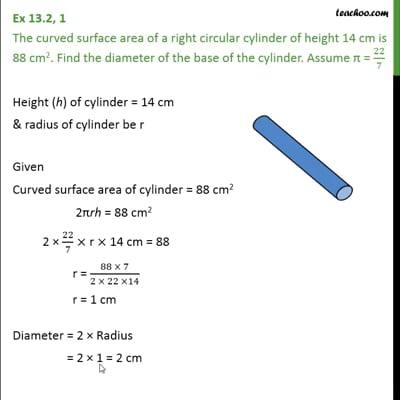Area Of Cylinder

Chapter 13 Class 9 Surface Areas and Volumes
Concept wiseThis video is only available for Teachoo black users

Introducing your new favourite teacher - Teachoo Black, at only ₹83 per month

### Transcript

Ex 13.2, 1 The curved surface area of a right circular cylinder of height 14 cm is 88 cm2. Find the diameter of the base of the cylinder. Assume = 22/7 Height (h) of cylinder = 14 cm & radius of cylinder be r Given Curved surface area of cylinder = 88 cm2 2 rh = 88 cm2 2 22/7 r 14 cm = 88 r = (88 7)/(2 22 14) r = 1 cm Diameter = 2 Radius = 2 1 = 2 cm Therefore, the diameter of the base of the cylinder is 2 cm.